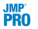Predictive and Specialized Modeling > Gaussian Process > Launch the Gaussian Process Platform
Publication date: 08/13/2020

# Launch the Gaussian Process Platform

Launch the Gaussian Process platform by selecting Analyze > Specialized Modeling > Gaussian Process.

Figure 16.4 Gaussian Process Launch WindowY

Assigns the continuous columns to analyze.

X

Assigns the columns to use as explanatory variables. Categorical variables are allowed in JMP Pro when the Fast GASP option is specified.

Estimate Nugget Parameter

Introduces a ridge parameter into the estimation procedure. A ridge parameter is useful if there is noise or randomness in the response, and you want the prediction model to smooth over the noise instead of perfectly interpolating.Fast GASP

Option to use the Fast GASP algorithm. Fast GASP breaks the Gaussian process model into small pieces (called blocks) to speed computation time. Blocks allow for the use of multiple CPUs and parallel processing.

Note: When there are more than 2,500 observations, the Fast GASP algorithm is required.

Correlation Type

Choose the correlation structure for the model. The platform fits a spatial correlation model to the data, where the correlation of the response between two observations decreases as the values of the independent variables become more distant.

Gaussian

Restricts the correlation between two points to always be nonzero, no matter the distance between the points.

Cubic

Allows the correlation between two points to be zero for points that are far enough apart. This method is a generalization of a cubic spline.The Fast GASP algorithm does not support the cubic correlation function.

Minimum Theta Value

Sets the minimum theta value to use in the fitted model. The default is 0. The theta values are analogous to a slope parameter in regular regression models. Small theta values indicate that a variable has little influence on the predicted values.Block Size

The number of observations in each computational block used by the Fast GASP algorithm. There must be at least 25 observations per block and a maximum of the number of rows in the data set up to a maximum of 2,500.